nextnano.com  GUI: nextnanomat  Tool: nextnano++  Tool: nextnano³  Tool: nextnano.NEGF  Download | Search | Copyright | News | Publications  * password protected nextnano³ software1D AlAs QW crossover I

# nextnano3 - Tutorial

## Strained AlAs QW - Crossover of ground states

Author: Stefan Birner

If you want to obtain the input files that are used within this tutorial, please contact stefan.birner@nextnano.de.
```-> 1DAlAs_QW_4nm_001.in -> 1DAlAs_QW_8nm_001.in -> 1DAlAs_QW_4nm_011.in -> 1DAlAs_QW_8nm_011.in -> 1DAlAs_QW_4nm_111.in  ```

## Strained AlAs QW along  - Crossover of ground states

```-> 1DAlAs_QW_4nm_001.in -> 1DAlAs_QW_8nm_001.in```

Note: Please also have a look at this tutorial: AlAs QW crossover II

### Conduction band edges and wave functions

The following figures show the conduction band edges at the X point for AlAs(001) QWs embedded within GaAs.
The square of the wave functions (psi2) - shifted by their energies - are shown for a 4 nm and 8 nm quantum well.
The X band in AlAs is split due to compressive strain with respect to the GaAs(001) substrate.
The Xxy band is two-fold degenerate (2 valleys at the X point).``` ==> ```mDOS = (ml mt)1/2
The Xz band is non-degenerate (1 valley at the X point).
`      ==> `mDOS = (mt mt)1/2 = mt
The Xz and Xxy valleys are separated due to strain by 21.6 meV.
The GaAs barriers are unstrained.
Only the two lowest confined wave functions are shown.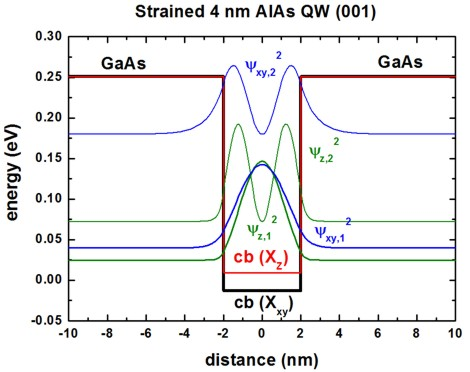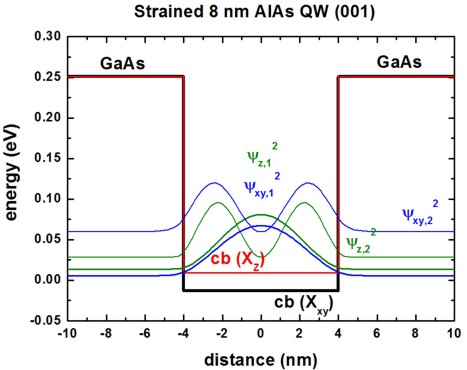The longitudinal mass ml is heavier in AlAs than the transverse masses mt.
Consequently, one expects the ground state to be determined by the heavier longitudinal mass.
However, this is true only for QWs smaller than 6 nm.
For larger QW widths, the ground state is due to the other two valleys because here, the fact that these conduction band edges are the lowest in energy dominates.

The following figure shows the energy spectrum of a strained AlAs(001) QW as a function of QW width.
Once can clearly see the crossing of the ground state at a QW width of ~6 nm.
For the 10 nm QW, 4 states of the Xxy valleys and 8 states of the Xz valleys are confined in the quantum well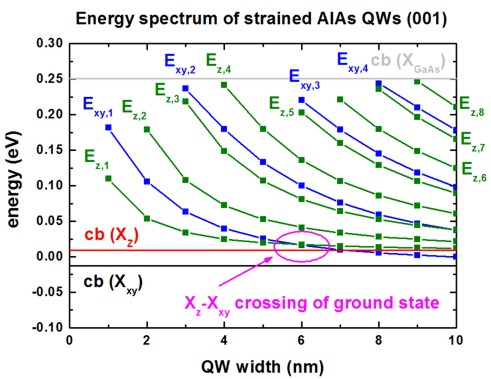### Material parameters

• ``` \$numeric-control  ...  lattice-constants-temp-coeff-on = yes ! ```temperature dependent lattice constants

``` \$global-parameters  lattice-temperature = 1d0 ! [K] ```1 Kelvin

T = 1 K, i.e. lattice constants are calculated for 1 K:
`==> `GaAs: 0.5642 nm
`==> `AlAs:  0.5652 nm

These calculated values can be found in this file:``` lattice_constants1D.dat  ```
• X band conduction band offset (CBO) GaAs/AlAs (unstrained): 0.251 eV = `3.441 - 3.190`

GaAs```  conduction-band-energies = ...  ...  3.441d0 ! [eV] Gamma, L, X ```AlAs```  conduction-band-energies = ...  ...  3.190d0 ! [eV] Gamma, L, X ```We shift both band edges by` -3.190 ` so that the unstrained AlAs band edge is located at 0 eV.```  band-shift               = -3.190d0          ! [eV]  ```
• GaAs``` conduction-band-masses = ...                  ! [m0] Gamma cb1 - ```1st principal axis, 2nd, 3rd```                          ...                  ! [m0] L     cb2 - ```1st principal axis, 2nd, 3rd``` - ml mt mt                          1.3d0  0.23d0 0.23d0 ! [m0] X     cb3 - ```1st principal axis, 2nd, 3rd``` - ml mt mt ```AlAs``` conduction-band-masses = ...                  ! [m0] Gamma cb1 - ```1st principal axis, 2nd, 3rd```                          ...                  ! [m0] L     cb2 - ```1st principal axis, 2nd, 3rd``` - ml mt mt                          0.97d0 0.22d0 0.22d0 ! [m0] X     cb3 - ```1st principal axis, 2nd, 3rd``` - ml mt mt ```
The mass tensor at the X conduction band edge is described with a longitudinal mass and two transverse masses (ellipsoidal mass tensor).

## Strained AlAs QW along  - Crossover of ground states

```-> 1DAlAs_QW_4nm_011.in -> 1DAlAs_QW_8nm_011.in```

Here, we grow the AlAs QW along the  direction.
This will affect the strain tensor, the shifts and splittings of the X conduction band edges and the orientation of the effective mass tensors of the different valleys.

``` \$domain-coordinates !   ...   growth-coordinate-axis = 0 0 1 !   !++++++++++++++++++++ (011) surface +++++++++++++++++++++++++++++++++++++++++    hkl-x-direction-zb = 1 0  0 ! ```along the   direction in the crystal coordinate system```    hkl-y-direction-zb = 0 1 -1 ! ``` along the [01-1] direction in the crystal coordinate system```   !hkl-z-direction-zb = 0 1  1 ! ``` along the   direction in the crystal coordinate system```   !++++++++++++++++++++ (011) surface +++++++++++++++++++++++++++++++++++++++++  ```

### Conduction band edges and wave functions

The following figures show the conduction band edges at the X point for AlAs(011) QWs embedded within GaAs.
The square of the wave functions (psi2) - shifted by their energies - are shown for a 4 nm and 8 nm quantum well.
The X band in AlAs is split due to compressive strain with respect to the GaAs(011) substrate.
The Xx band is not degenerate (1 valley at the X point).```      ==> ```mDOS = (ml mt)1/2
The Xyz band is two-fold degenerate (2 valleys at the X point).
` ==> `mDOS = (mt mt)1/2 = mt
The Xyz and Xx valleys are separated due to strain by 9.4 meV, so this is only half as much as for the (001) case.
The GaAs barriers are unstrained.
Only the two lowest confined wave functions are shown.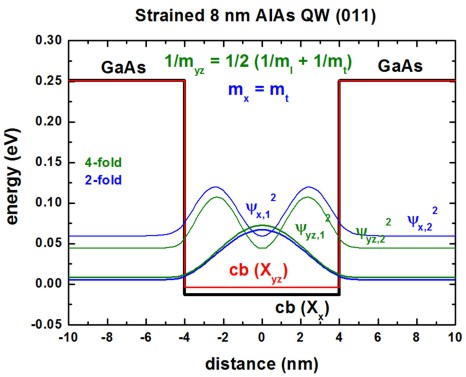We now have different mass tensors compared to the (001) QW.
For the confinement direction z, the following mass tensor components are relevant for the Schrödinger equation:
AlAs: cb(Xx):  1/mzz(011) = 1/mt = 1 / 0.22
AlAs: cb(Xyz): 1/mzz(011) = 1 / 2  ( 1/ml + 1/mt ) = 1 / 0.359

The mass for the two Xyz band edges is heavier than the transverse masses mt that is relevant for the Xyz band edge.
Consequently, one expects the ground state to be determined by the heavier mass.
However, this is true only for QWs smaller than 6 nm.
For larger QW widths, the ground state is due to the other valley because here, the fact that this conduction band edge is the lowest in energy dominates.

The following figure shows the energy spectrum of a strained AlAs(011) QW as a function of QW width.
Once can clearly see the crossing of the ground state at a QW width of ~6 nm.
For the 10 nm QW, 4 states of the Xx valley and 5 states of the Xyz valleys are confined in the quantum wellWhen comparing the mass tensors for (001) and (011), one can see that the mass tensor became more "isotropic" for the (011) QW (but it is still ellipsoidal).
This fact can also be seen in the energy spectrum.

## Strained AlAs QW along  - Crossover of ground states

`-> 1DAlAs_QW_4nm_111.in`

Note: For strained heterostructures grown along the  growth direction piezoelectric fields are present! This has been neglected in this tutorial.

The following figure shows the conduction band profile and the lowest two wave functions (psi2) for the 4 nm AlAs QW grown along the  direction.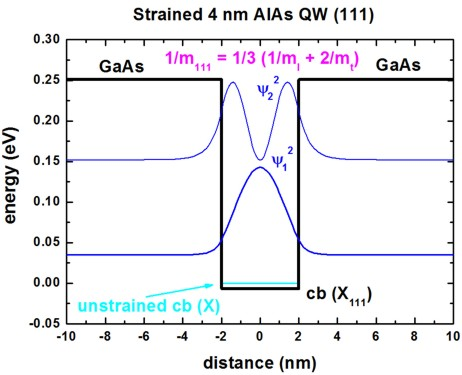For the  growth direction, the three X band edges are degenerate.
In that case a crossover cannot be observed as each eigenvalue is three-fold degenerate.

AlAs: cb(X111): 1/mzz(111) = 1 / 3  ( 1/ml + 2/mt ) = 1 / 0.296

The component 1/mzz for each grid point can be found in this file:
`Schroedinger_1band/mass_tensor1D_cb003_qc001_sg001_deg001.dat`

The following figure shows the relevant energy spectrum as a function of QW width.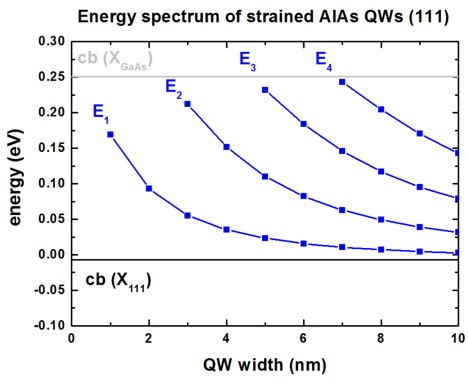• Please help us to improve our tutorial. Send comments to``` support [at] nextnano.com```.